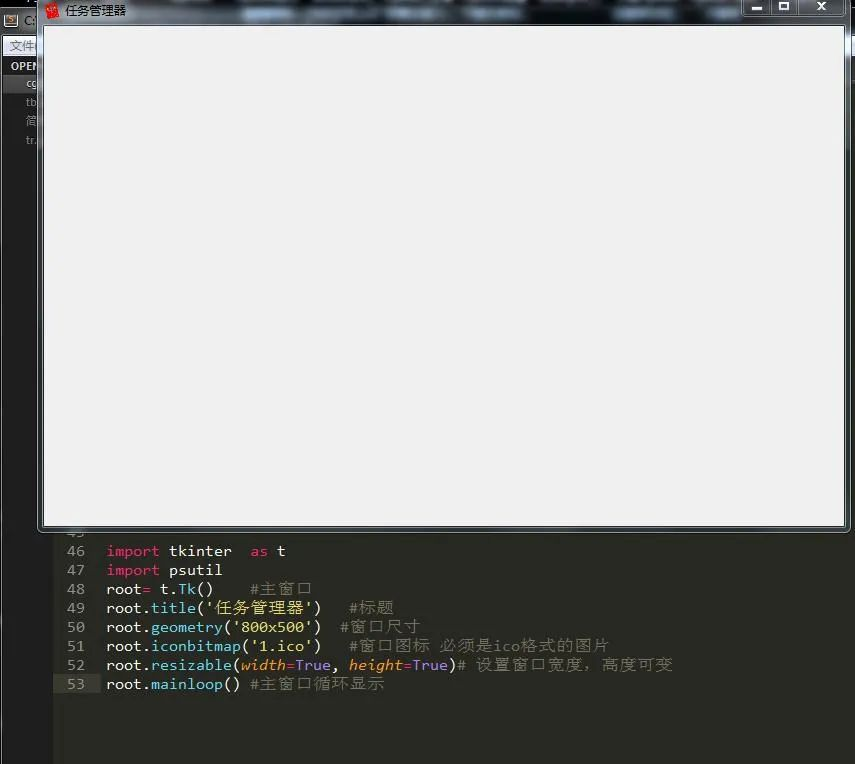##全国校区# 手把手教你用Python高仿一个任务管理器2021-07-23# 二、实现步骤

1、编写主界面2、编写菜单栏

1）首先我们需要创建一个主菜单，然后将各个子菜单装进去：

`m=t.Menu(root)`

2）创建各个子菜单：

```#文件菜单 file=t.Menu(m,tearoff=False)
ii.set(1)
iv.set(1)

3）将菜单添加到主界面配置中

`root.configure(menu=m)`3、界面中的功能

1）编写按钮

```b1=t.Button(root,text='应用程序',command=yy)
b2=t.Button(root,text='进程',command=jc)
b3=t.Button(root,text='服务',command=fw)
b4=t.Button(root,text='性能',command=xn)
b5=t.Button(root,text='联网',command=lw)
b6=t.Button(root,text='用户',command=yh) #定位 b1.place(x=10,y=15,height=20,width=60)
b2.place(x=70,y=15,height=20,width=60)
b3.place(x=130,y=15,height=20,width=60)
b4.place(x=190,y=15,height=20,width=60)
b5.place(x=250,y=15,height=20,width=60)
b6.place(x=310,y=15,height=20,width=60)```

2）编写多行文本框

```text=t.Text(root,width=100,height=40)
text.place(x=10,y=36)```

3）编写函数实现简单功能

`def yy(): text.delete(1.0,'end') text.insert('insert','yy') def jc(): text.delete(1.0,'end') text.insert('insert','jc') def fw(): text.delete(1.0,'end') text.insert('insert','fw') def xn(): text.delete(1.0,'end') text.insert('insert','xn') def lw(): text.delete(1.0,'end') text.insert('insert','lw') def yh(): text.delete(1.0,'end') text.insert('insert','yh')`

4）编写下面的进程数，CPU使用率，物理内存

```t1=t.Label(text='进程数：')
t2=t.Label(text='CPU 使用率：')
t3=t.Label(text='物理内存：')
t1.place(x=10,y=580,width=120)
t2.place(x=150,y=580,width=120)
t3.place(x=300,y=580,width=120)```

5）给多行文本框添加滚动条

1.指定该组件的yscrollbarcommand参数为Scrollbar的set()方法

2.指定Scrollbar 的 command 参数为该组件的 yview() 方法

```sb=t.Scrollbar(root)
sb.pack(side='left',fill='y')
text=t.Text(root,width=100,height=40)
text.place(x=10,y=36)
sb.config(command=text.yview) #文本框内容随滚动条滚动 text.config(yscrollcommand=sb.set(0.1,0.3)) #Y轴填充```

6）添加状态栏文本标签

`t1=t.Label(text='') t2=t.Label(text='') t3=t.Label(text='')`

（注：这里只是隐藏部件，万不可用destroy销毁部件）

7）实现状态栏标签功能

```def jcs(): t1.configure(text='进程数：'+str(len(psutil.pids())))
root.after(3000,jcs) def cpu(): pp=str(ceil(psutil.cpu_percent(1)))
t2.configure(text='CPU 使用率：'+pp+'%')
root.after(1500,cpu) def wlnc(): f= psutil.virtual_memory().free #剩余内存 t=psutil.virtual_memory().total#总内存 wl= float(t-f)/float(t) #为使得最后值更精确，必须用float t3.configure(text='物理内存：'+str(floor(wl*100))+'%')
root.after(2000,wlnc)```

8）功能编写

1.编写应用程序选项

```text.insert('insert','进程号   '+'进程名      '+'  进程文件路径'+'n') for y in psutil.pids():
a=psutil.Process(y) if a.name()=='System Idle Process': continue else:
text.insert('insert',str(y)+'     '+a.name()+'   '+a.exe()+'nn')```

2.编写进程选项

```mm=os.popen('tasklist')

3.编写服务选项

```mm=os.popen('sc query type= service')

4.编写性能选项

```l1=t.Label(root,text='开机时间：')  tm=datetime.datetime.fromtimestamp(psutil.boot_time()).strftime("%Y-%m-%d %H:%M:%S")
l2=t.Label(root,text=str(tm))
l3=t.Label(root,text='当前时间：')
l4=t.Label(root,text='')
dq=time.strftime('%Y-%m-%d %H:%M:%S',time.localtime(time.time()))
l4.configure(text=str(dq))
l5=t.Label(root,text='物理内存使用情况(MB)：')
l6=t.Label(root,text='')
jh=psutil.virtual_memory() #物理内存 tt=int((jh.total)/1024/1024) #总量 us=int((jh.used)/1024/1024) #使用量 fr=int((jh.free)/1024/1024) #剩余量 l6.configure(text='总量：' + str(tt) +'n'+'使用：'+str(us) +'n'+'剩余：'+str(fr))
l7=t.Label(root,text='交换内存使用情况(MB)：')
l8=t.Label(root,text='')
hj=psutil.swap_memory() #交换内存 ht=int((hj.total)/1024/1024)
hu=int((hj.used)/1024/1024)
hf=int((hj.free)/1024/1024)
l8.configure(text='总量：' + str(ht) + '  '+'使用：'+str(hu) +'  '+'剩余：'+str(hf))
text.window_create('insert',window=l1) #添加组件到多行文本框 text.window_create('insert',window=l2)
text.insert('insert','nn')
text.window_create('insert',window=l3)
text.window_create('insert',window=l4)
text.insert('insert','nn')
text.window_create('insert',window=l5)
text.window_create('insert',window=l6)
text.insert('insert','nn')
text.window_create('insert',window=l7)
text.window_create('insert',window=l8)```

5.编写联网选项

```n = psutil.net_io_counters()
r=str(float(n.bytes_recv / 1024 / 1024))+'MB'
s= str(float(n.bytes_sent / 1024 / 1024))+'MB'
text.insert('insert','网卡接收流量: '+str(r)+'n'+'网卡发送流量：'+str(s)+'n')```

6.编写用户选项

```use='    用户'+'      '+'     状态'+'n' text.insert('insert',use) for y in psutil.users():
text.insert('2.0',str(y.name)+'  '+'运行中。。。。'+'n')```OK